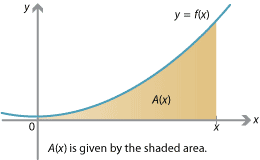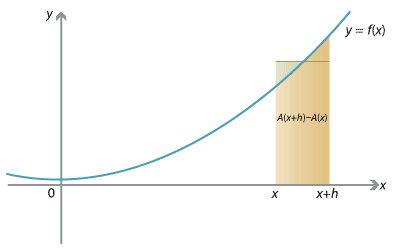## Content

### Area and the antiderivative

Let's return to our function $$f(x) = x^2 + 1$$, and our quest to find the area under the graph $$y=f(x)$$ between $$x=0$$ and $$x=5$$, i.e.,

$\int_0^5 (x^2 + 1) \; dx.$

As we've seen, we can estimate the area by many narrow rectangles, each over a subinterval of $$[0,5]$$, with width $$\Delta x$$ and height given by a value of $$f(x)$$. As we take the limit of more and more narrower and narrower rectangles, we get a better approximation to the area.

To calculate the exact area, we introduce an area function $$A$$. For any fixed number $$c > 0$$, let $$A(c)$$ be the area under the graph $$y=f(x)$$ between $$x=0$$ and $$x=c$$. So we can define the function $$A(x)$$ to be the area between 0 and $$x$$. Clearly $$A(0) = 0$$. We seek $$A(5)$$.Detailed description of diagram

The fundamental idea is to consider the derivative of the area function $$A$$. What is $$A'(x)$$?

In fact, $$A'(x) = f(x)$$. We can see this geometrically. To differentiate $$A(x)$$ from first principles, we consider

$\frac{A(x+h) - A(x)}{h}.$

Now $$A(x+h)$$ is the area under the graph up to $$x+h$$, and $$A(x)$$ is the area under the graph up to $$x$$. Thus $$A(x+h) - A(x)$$ is the area under the graph between $$x$$ and $$x+h$$.Detailed description of diagram

If you were to 'level off' the area under the graph between $$x$$ and $$x+h$$, you'd obtain a rectangle (as shown in the graph above) with width $$h$$ and height equal to the average value of $$f$$; dividing the area by $$h$$ gives the average value:

\begin{align*} \frac{A(x+h)-A(x)}{h} &= \frac{\text{ area of rectangle }}{\text{ width of rectangle }} \\ &= \text{ height of rectangle } \\ &= \text{ average value of $$f$$ over the interval $$[x, x+h]$$.} \end{align*}

If $$h$$ becomes very small, then the interval $$[x,x+h]$$ approaches the single point $$x$$. And the average value of $$f$$ over this interval must approach $$f(x)$$. So we have

$\lim_{h \to 0} \frac{A(x+h)-A(x)}{h} = f(x).$

In other words,

$A'(x) = f(x),$

and $$A(x)$$ is an antiderivative of $$f(x)$$.

Returning again to our example $$f(x) = x^2 + 1$$, what then is the area function $$A(x)$$? Its derivative must be $$f(x) = x^2 + 1$$. We're looking for a function $$A$$ such that

$A'(x) = x^2 + 1 \qquad \text{and} \qquad A(0)= 0.$

Thinking back to derivatives, we can see that the antiderivative

$A(x) = \frac{1}{3} x^3 + x$

satisfies these criteria. Hence the total area is

$A(5) = \frac{125}{3} + 5 = \frac{140}{3} = 46 \frac{2}{3},$

confirming our earlier estimates. We can write this as

$\int_0^5 f(x) \; dx = A(5) - A(0) = \frac{140}{3}.$

Next page - Content - Antiderivatives and indefinite integrals